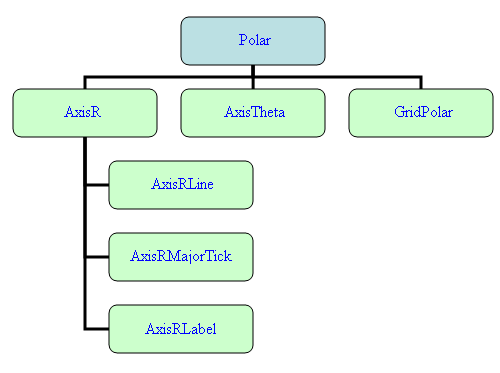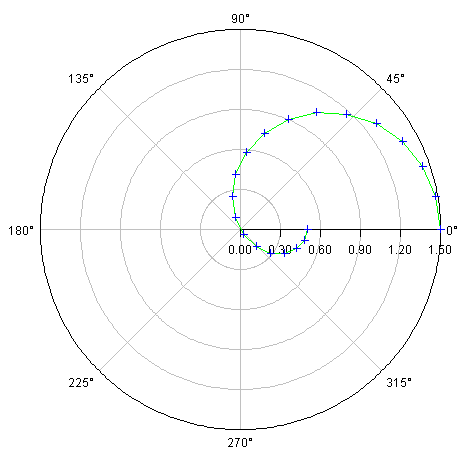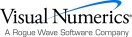## Polar Plot

The class Polar is used to plot (r,theta) data, where r is the distance from the origin and theta is the angle from the positive x-axis. Data node (x, y) values are treated as if they were (r,theta) values.

When a Polar node is created, a subtree of other nodes is created. These automatically created nodes are shown in the following diagram.AxisR is the r-axis node. This is drawn along the positive x-axis. This node has three subnodes: AxisRLine for the actual line, AxisRMajorTick for the tick marks along the line and AxisRLabel for the tick mark labels.

The drawing of the AxisRLine and the AxisRMajorTick is controlled by the Line Attributes. The drawing of the AxisRLabel is controlled by the Text Attributes.

The Window attribute in AxisR specifies the length of the r-axis. Since the r-axis range is
[0, rmax], the Window attribute for this node is the rmax value. Autoscaling is used to automatically determine rmax from the data. Autoscaling can be turned off. See Autoscale for more details.

AxisTheta  is the theta-axis node. It is drawn as a circle around the plot. The drawing of the circle itself is controlled by the line attributes. The drawing of the axis labels is controlled by the text attributes.

The Window attribute in the AxisTheta node contains the angular range, in radians. Its default value is [0,2π]. The Window attribute can be explicitly set to change the range, but autoscaling is not available to determine the angular range.

GridPolar is the plot grid. It consists of both radial and circular lines. The drawing of these lines is controlled by the line attributes. By default, these lines are drawn and are light gray.

### Example

The function r = 0.5 + cos(q), for 0 <= q <= p is plotted.using Imsl.Chart2D;

using System.Drawing;

using System;

public class SamplePolar : FrameChart {

public SamplePolar() {

Chart chart = this.Chart;

Polar axis = new Polar(chart);

double[] r = new double;

double[] theta = new double[r.Length];

for (int k = 0; k < r.Length; k++) {

theta[k] = Math.PI*k/(r.Length-1);

r[k] = 0.5 + Math.Cos(theta[k]);

}

Data data = new Data(axis, r, theta);

data.DataType = Data.DATA_TYPE_MARKER | Data.DATA_TYPE_LINE;

data.LineColor = Color.Lime;

data.MarkerColor = Color.Blue;

}

public static void Main(string[] argv) {

System.Windows.Forms.Application.Run(new SamplePolar());

}

}Visual Numerics - Developers of IMSL and PV-WAVEhttp://www.vni.com/PHONE: 713.784.3131FAX:713.781.9260